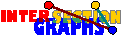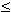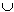### Interval bigraphs

Interval digraphs--- intersection bigraphs of intervals of the real line---have been introduced in [SDRW89]. ---we deal here with the more general bigraph model. See an example (dancing club).

There is a notion similiar to that of asteroidal triples in graphs: An asteroidal triple of edges is formed by three edges where any two are connected by a path avoiding vertices and neighbors of the third edge.[M97]: No interval bigraph contains an asteroidal triple of edges
 Proof: Assume an interval bigraph contains an asteroidal triple u(1)w(1), u(2)w(2), u(3)w(3) of edges. Then Su(i)Tw(i),i=1,2,3, are three disjoint intervals in R. Assume Su(i)Tw(i) lies between the other two. But then, for every path from u(1)w(1) to u(2)w(2), some representator must intersect Su(2)Tw(2), a contradiction.

This analogue of asteroidal-triple-freeness of interval graphs surprisingly implies an analogue of chordality of interval graphs:[M97]: Interval bigraphs do not contain induced cycles of length 6 or more.

There follows that for this model the converse of the above proposition holds:A graph G is an interval graph if and only if B(G) is an interval bigraph.
 Proof: Assume G is no interval graph. If G contains an asteroidal triple x,y,z, then we get an asteroidal triple xx´, yy´, zz´ of edges in B(G). Thus B(G) is no interval bigraph. Otherwise, by Lekkerkerker and Boland´s characterization, G must contain some induced Cp with p > 3. But then B(G) contains some induced Cm with m > 5, and again B(G) is no interval bigraph.

Therefore, recognizing interval bigraphs is at least as difficult than recognizing interval graphs. The difficulty of recognizing interval bigraphs may be due to the fact that, although intervals of the real line have the Helly-property, the bicliques, i.e. inclusion-maximal complete bipartite subgraphs, are no longer necessarily star graphs. All one can show that for every biclique U*W, eitheruU Su orwW Tw must be nonempty. Thus finding out the star graphs seems to be a lot more difficult in this model than for intersection graphs. The only known efficient method for recognizing interval bigraphs doesn`t use star graphs but separators.[M97]: Interval bigraphs can be recognized in time O(nm6(n+m)log n), where n and m are number of vertices and edges.Is there some quicker or simpler recognition algorithm for interval bigraphs? Is there one which works by revealing the star graphs?

For proper interval bigraphs we require that all intervals Sx are pairwise incomparable, as well as all Ty. Proper interval bigraphs have a nice characterization which again uses the order of intervals on R:[S96]: A bipartite graph is a proper interval bigraph if and only if it is a permutation graph.
 Proof: Let B be the intersection bigraph of the two families of intervals ([ax,bx]/ xU) and ([cy,dy]/ yW). We construct lines between the x-axis and the line y=1 by defining Rx to be the line from (ax,0) to (bx,1), for xU and otherwise, if xW, let Rx be the line from (cy,1) to (dy,0). Since avaz < bzbv is impossible for distinct v,zU, Rv and Rz are disjoint, and the same if both v and z lie in W. On the other hand, for xU and yW, RxRy is nonempty if and only if SxTy is nonempty. Assume conversely that the bipartite graph B with bipartition UW is a permutation graph, i.e. the intersection graph of a family (Rz/zUW) of straight lines between two parallel lines. It is not too difficult to show that this representation can be changed in such a way that for every xW, the lower endpoint sits to the left of the upper endpoint, and conversely for every yW. The remainder of the proof, the construction of the intervals, reverses the construction in the first part of the proof.

Therefore, proper interval bigraphs can be recognized in time O(n2).

Do you want to visit also line bigraphs, or go back to the start page for intersection graphs?

Erich Prisner